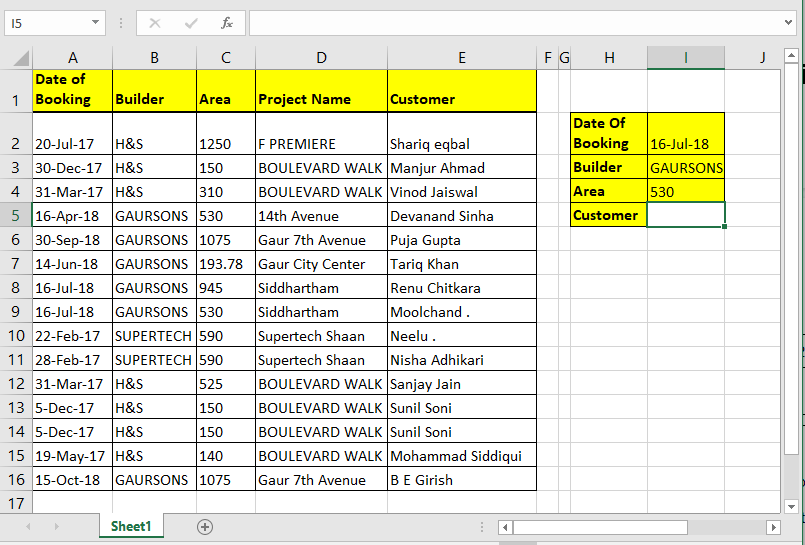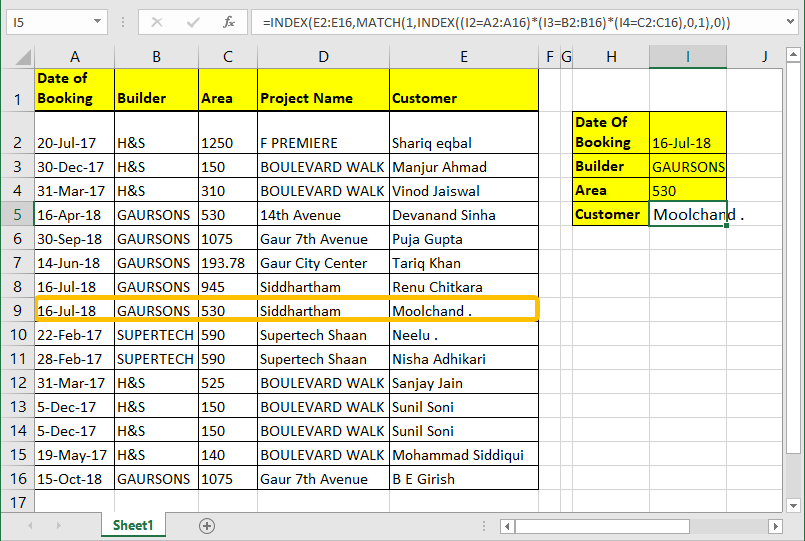# How to Retrieve a Price from a List that Matches both Category and Item Criteria in Excel

In this article, we will learn How to Retrieve a Price from a List that Matches both Category and Item Criteria in Excel.

Scenario:

It's easy to find a value with one criteria in a table, for that We could simply use VLOOKUP. But when you don’t have a single column criteria in your data and need to find through multiple columns criteria to match a value, VLOOKUP doesn’t help.

Generic Formula for both category and Item

 =INDEX(lookup_range,MATCH(1,INDEX((item1=item_range) * (category2=category_range),0,1),0))

lookup_range: It's the range from which you want to retrieve value.

Criteria1, Criteria2, Criteria N: These are the criteria you want to match in range1, range2 and Range N. You can have upto 270 criteria - range pairs.

Range1, range2, rangeN : These are the ranges in which you will match your respective criteria

Example :

All of these might be confusing to understand. Let's understand how to use the function using an example. Here we have a data table. I want to pull the Name of the customer using Date of Booking, Builder and Area. So here I have three criteria and one lookup range.Write this formula in cell I4 hit enter.

 =INDEX(E2:E16,MATCH(1,INDEX((I1=A2:A16)*(I2=B2:B16)*(I3=C2:C16),0,1),0))We already know how INDEX and MATCH function work in EXCEL, so not going to explain that here. We will talk about the trick we used here.

(I1=A2:A16)*(I2=B2:B16)*(I3=C2:C16): The main part is this. Each part of this statement returns an array of true false.

When boolean values are multiplied they return an array of 0 and 1. Multiplication works as an AND operator. Hense when all value are true only then it returns 1 else 0

(I1=A2:A16)*(I2=B2:B16)*(I3=C2:C16) This altogether will return

Which will translate into

 {0;0;0;0;0;0;0;1;0;0;0;0;0;0;0}

INDEX((I1=A2:A16)*(I2=B2:B16)*(I3=C2:C16),0,1): INDEX Function will return the same array ({0;0;0;0;0;0;0;1;0;0;0;0;0;0;0}) to MATCH function as lookup array.

MATCH(1,INDEX((I1=A2:A16)*(I2=B2:B16)*(I3=C2:C16),0,1): MATCH function will look for 1 in array  {0;0;0;0;0;0;0;1;0;0;0;0;0;0;0}. And will return the index number of the first 1 found in the array. Which is 8 here.

INDEX(E2:E16,MATCH(1,INDEX((I1=A2:A16)*(I2=B2:B16)*(I3=C2:C16),0,1),0)): Finally, INDEX will return the value from the given range (E2:E16) at found index (8).

# Array formula

If you can hit CTRL + SHIFT + ENTER consequently then you can eliminate the inner INDEX function. Just write this formula and hit CTRL + SHIFT + ENTER.

Formula

 =INDEX(E2:E16,MATCH(1,(I1=A2:A16)*(I2=B2:B16)*(I3=C2:C16),0))

Generic Array Formula for Multiple Criteria Lookup

 =INDEX(lookup_range,MATCH(1,(criteria1 =range1)*(criteria2=range2)*(criteriaN=rangeN),0))

Here are all the observational notes using the formula in Excel
Notes :

1. Use Vlookup if you have one criteria to match, it's common practice.
2. You can add more rows and columns in the lookup array.
3. Text arguments must be given within quotes ("").
4. The VLOOKUP table must have the lookup_array in the leftmost or the first column.
5. The col index cannot be 1 as it is the lookup array
6. 0 argument is used for the exact match value. Use 1 for the approximate match value.
7. The function returns #N/A error, if look up value is not found in the lookup array. So catch the error, if necessary.

Hope this article about How to Retrieve a Price from a List that Matches both Category and Item Criteria in Excel is explanatory. Find more articles on calculating values and related Excel formulas here. If you liked our blogs, share them with your friends on Facebook. And also you can follow us on Twitter and Facebook. We would love to hear from you, do let us know how we can improve, complement or innovate our work and make it better for you. Write to us at info@exceltip.com.

Related Articles :

How to Retrieve Latest Price in Excel : It is common to update prices in any business and using the latest prices for any purchase or sales is a must. To retrieve the latest price from a list in Excel we use the LOOKUP function. The LOOKUP function fetches the latest price.

VLOOKUP function to calculate grade in Excel : To calculate grades IF and IFS are not the only functions that you can use. The VLOOKUP is more efficient and dynamic for such conditional calculations.To calculate grades using VLOOKUP we can use this formula.

17 Things About Excel VLOOKUP : VLOOKUP is most commonly used for retrieving matched values but VLOOKUP can do a lot more than this. Here are 17 things about VLOOKUP that you should know to use effectively.

LOOKUP the First Text from a List in Excel : The VLOOKUP function works fine with wildcard characters. We can use this to extract the first text value from a given list in excel. Here is the generic formula.

LOOKUP date with last value in list : To retrieve the date that contains the last value we use the LOOKUP function. This function checks for the cell that contains the last value in a vector and then uses that reference to return the date.

Popular Articles :

How to use the VLOOKUP Function in Excel : This is one of the most used and popular functions of excel that is used to lookup value from different ranges and sheets.

How to use the IF Function in Excel : The IF statement in Excel checks the condition and returns a specific value if the condition is TRUE or returns another specific value if FALSE.

How to use the SUMIF Function in Excel : This is another dashboard essential function. This helps you sum up values on specific conditions.

How to use the COUNTIF Function in Excel : Count values with conditions using this amazing function. You don't need to filter your data to count specific values. Countif function is essential to prepare your dashboard.

1.If you have low and high price.
How to make lookup select it self price which is connected to one customer.
If customer one have low price.

2.How to revise the formula for a price list in another sheet? Thanks in advance!

•Simply add the sheet name with ! before the range and it will be done. for example, if your data in sheet2 the formula would be:
{=INDEX(\$C\$2:\$C\$11,MATCH(E2&F2,Sheet2!\$A\$2:\$A\$11&Sheet2!\$B\$2:\$B\$11,0))}

3.Rudi van der Westhuyzen

The example above does not work in Excel Office 365. What should the revised formula be?

•Rudi van der Westhuyzen

Fixed...found the fault Thank you

4.Here, I want if I enter latest price of laptop A in A2 then in G2 there's automatically updated the new price.Like if I enter in A2 the price of Laptop A 500 so in G2 excel should automatically update the new price .. Is it possible....??

Terms and Conditions of use

The applications/code on this site are distributed as is and without warranties or liability. In no event shall the owner of the copyrights, or the authors of the applications/code be liable for any loss of profit, any problems or any damage resulting from the use or evaluation of the applications/code.